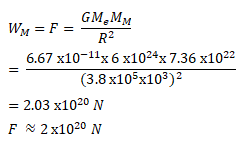Deepak Scored 45->99%ile with Bounce Back Crack Course. You can do it too!

# The force with which the earth attracts an object

Question:

The force with which the earth attracts an object is called the weight of the object.

Calculate the weight of the moon from the following data: The universal constant of gravitation $G=6.67 \times 10^{-11} \mathrm{~N}-\mathrm{m}^{2} / \mathrm{kg}$, mass of the moon $=7.36 \times 10^{22} \mathrm{~kg}$, mass of the earth $=6 \times 10^{24} \mathrm{~kg} \mathrm{~ a n d ~ t h e ~ d i s t a n c e ~ b e t w e e n ~ t h e ~ e a r t h ~ a n d ~ t h e ~ m o o n = 3 . 8}$ $\mathrm{km}$.

Solution: